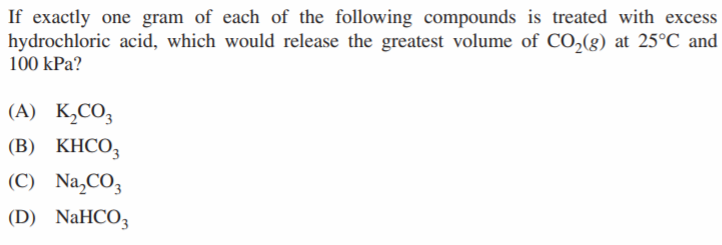# Chemistry Preliminary Exam

All questions for multiple choice are taken from Old HSC Exams, covering new syllabus Preliminary Chemistry content.

15 marks – 25 mins

Questions.

Question 1.

Question 2.

Question 3.

Question 4.

Question 5.

Question 6.

Question 7.

C6H12O6 = Glucose, fermentation converts glucose into ethanol and carbon dioxide

Question 8.

Question 9.

Question 10.Question 11.

Question 12.

Question 13.

Question 14.

Question 15.

Question 1.

D, chloroethene

Question 2.

C

Question 3.

D

Question 4.

D

Question 5.

B & C is complete combustion, D is not balanced

Question 6.

A

Question 7.

C6H12O6 = Glucose, fermentation converts glucose into ethanol and carbon dioxide

D

Question 8.

A

Question 9.

A

Question 10.If treating with HCl → H+ + Cl

Cation-carbonate + HCl →  cation-Cl+ H2O + CO2

D → lowest molar mass – most moles per gram

Question 11.

Ethanol = C2H5OH

1000 g of ethanol * (1 mol / (12.01*2+1.008*6+16.00) g) * (1360 kJ / 1 mol) * (1 km/ 2270 kJ)

B

Question 12.

C

Question 13.

A

Question 14.

B

Question 15.

• Lower activation energy, increase the rate of reaction

A